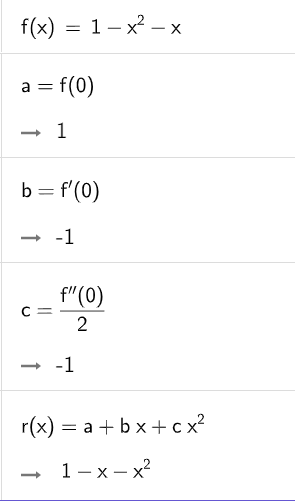# Polynomial in ascending power of x

Alex shared this question 2 years ago

I have a polynomial, say a random polynomial of degree 3. Is there a way to turn it into another equivalent polynomial in ascending powers of x?

Thanks.see attached file1

with text ? (no other polynomial)1

But when some of the coefficients are zero or one, your expression is not good enough for me.

When f(x)=x^3-x+4, I need another polynomial g(x)=4-x+x^3. Nothing more, nothing less.

Many thanks,

Alex1

see attached file

Files: aha.ggb1

Sorry your method seems work only in CAS environment. In normal Graphic View, the zeros and ones still appear. I try to avoid CAS methods like Simplify, Expand, etc.

See attached file.

Thanks anyway.

Files: aha2.ggb1

is TaylorPolynomial(f, 0, Degree(f)) enough?1

Not exactly. Thanks.1

más detalles corren por cuenta del proximo autor

Files: foro.ggb1

Maybe something like that1

see attached file1

Thanks. But Simplify() invokes CAS, which is not preferred.1

me gusta este ultimo1

but I agree that Jozef's answer is better :)1

Thanks. This is nearly perfect, except when a=b=0 and c=5, it gives result 0+5x^2 instead of 5x^2.1

So...1

Hope other users can benefit.1

or If(a^2+b^2==0,Polynomial(c x^2),r(x))

but it works only for quadratic function